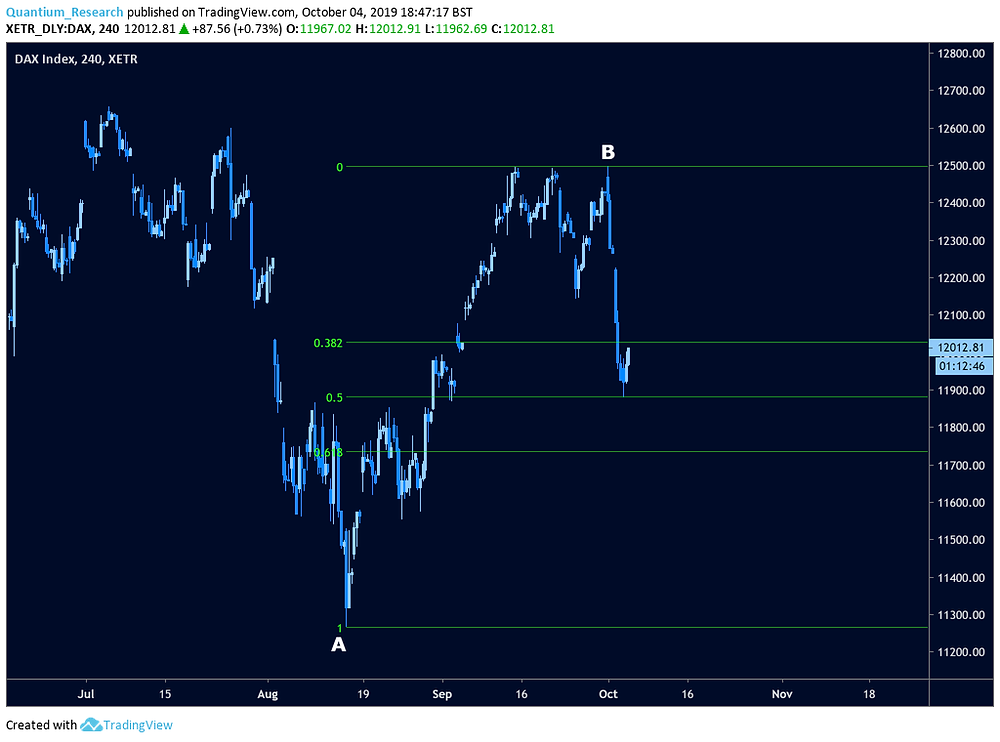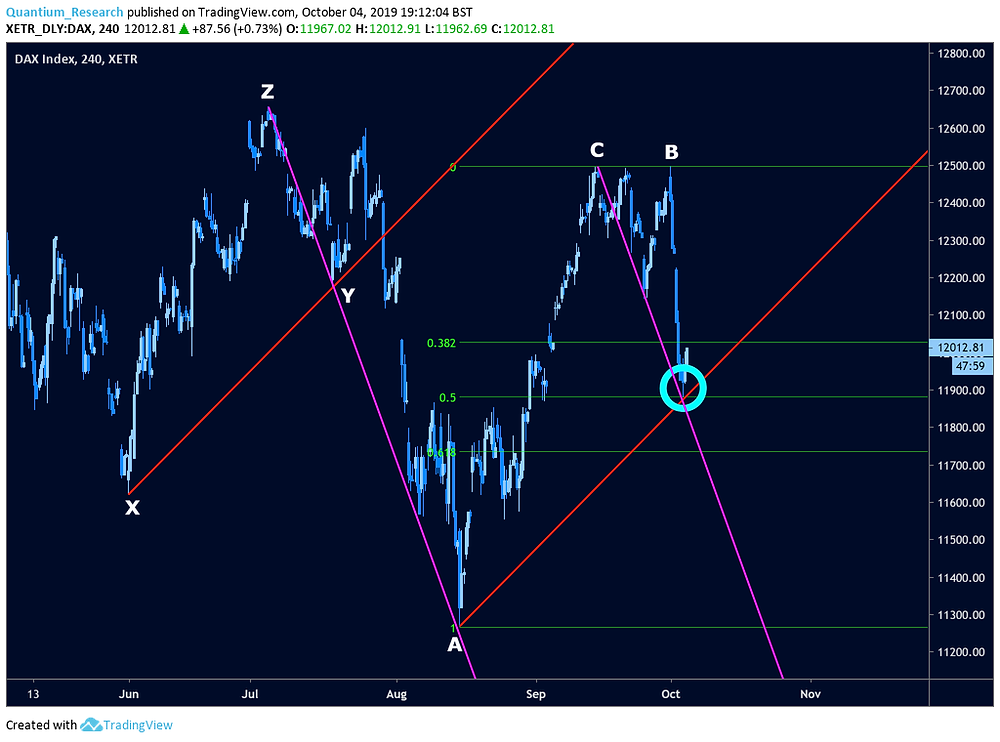Search

# The German DAX Could Be Primed For A Reversal

The German DAX has retraced into an interesting cluster of support which is worth being aware of. Understand the broad context of the market in the 4-hourly chart in figure 1. Recently, the market has risen into a consolidatory phase which produced a triple top. The third top was followed by a sharp retracement and that’s where the market stands at the time of writing.Figure 1

In determining levels where this retracement may end, firstly consider the Fibonacci division shown in figure 2. These are the green horizontal levels.Figure 2

The range is defined as the vertical distance between the point labelled ‘A’ and the point labelled ‘B’. Clearly, the market retraced as far as the 0.5 level and found some support there. It has started to rise but hasn’t yet reached the 0.382 level again. It’s possible that the retracement has completed as a 50% retracement and the prior uptrend will resume. It’s also possible that the support from the 0.5 level will only be enough for a minor bounce, after which the downward move will continue, perhaps as far as the 0.618 level or more. Further investigation can give a clearer picture.

The next point is based on the concept of angular symmetry. Observe the angle of ascent between the low at point X and the secondary low at point Y in figure 3. They are connected by a red solid line which is projected out into empty space.Figure 3

When that line is copied and, crucially, the gradient is kept constant and projected from the low at point A, the resulting line intersects the 0.5 retracement level at exactly where the market reversed in the cyan circle. This is another source of support, adding to the strength of the price barrier.

A similarly interesting result is obtained by applying a similar method to a different range in figure 4. The angle of descent is taken between point Z and point A. Notice that this is the range from a high to a low rather than a low to a low. This means the first purple line is representing the average gradient of the whole move between points Z and A.Figure 4

As before, when that line is copied exactly and projected from a different point, this time the high at C, the resulting line intersects at the same point as before in the cyan circle. This degree of confluence is already creating a compelling case for a reversal from the cyan circle.

Moving onto figure 5, look at the final upward swing before the retracement. This is the range between the low at D (just before the 3rd peak of the triple top) and the high at B.Figure 5

When Fibonacci divisions are applied to this range, notice that the 1.618 extension coincides exactly with the closing price of the lowest candlestick so far. Also inside the cyan circle, this adds even more support. Horizontal and diagonal overlays from four different sources now are all indicative of a reversal coming from the cyan circle.

An important observation is that each of the overlays shown so far could have been constructed long before the market came close to the cyan circle. As soon as the high at B was in place, this analysis could have been done and one could have been aware of the potential support coming from this area.

With this degree of confluence, it is very likely that this will be a very strong support level from which there will be a significant reversal which, it appears, has already begun. If, instead, the market falls below the 50% horizontal division of AB, the downward move will have shown signs of substantial strength and may have much more downside potential.

As a final thought, see figure 6. The vertical purple dashed lines divide the recent price action into two sections: the upward progressive section, and the downward corrective section.Figure 6

Assume that the price will continue to rise and that the retracement is complete. In that case, the whole cycle can be thought of as beginning at the low at A and ending in the cyan circle. This whole cycle would have lasted for 106 candlesticks. Of those, 65 were spent in the uptrend section which lasted until the high at C. That means that ((65/106) x 100)% of the time was spent in the uptrend. This comes out to be 61.3% which is roughly the Golden Ratio, phi, expressed as a percentage (61.8%).